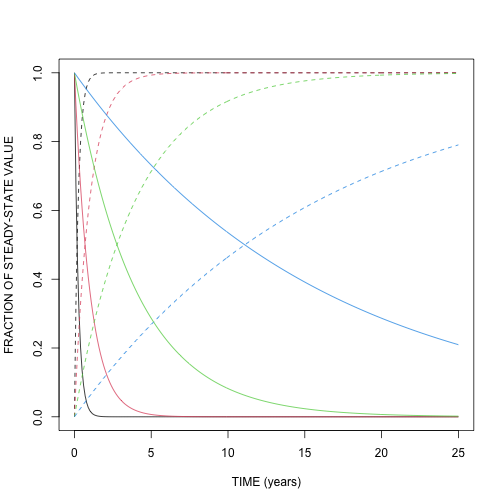In 1963, J. Olson published a very influential paper describing a differential equation for organic matter cycling in terrestrial ecosystems:

$\frac{dX}{dt} = L - k \cdot X,$ where the change in mass over time dX/dt is the difference between a steady income L and the loss rate kX, where k is the fractional loss rate. He calculated the analytical solution of this model to interpret the processes of C release and accumulation in ecosystems. In his example, release of organic matter was similar to litter decay in the absence of additional litter inputs, and it is given by the expression

$X = X_0 e^{(-k \cdot t)}.$

The accumulation of organic matter when inputs and outputs occur simultaneously, was expressed by Olson as

$X = \frac{L}{k} (1 - e^{-k \cdot t}),$

where L/k is the steady-state organic matter value.

As an example, Olson used different values of k to show how accumulation and release may differ among different ecosystems. He proposed that values of k = 4 are typical for tropical forests in Africa, and k = 1 for Andean forests in Colombia. For pine forests in the southeast of the US, he proposed a value of k = ¼, and for the same forest in Minnesota k =1/16.

We can easily implement Olson's model in SoilR using the function OnepModel. In this example, we will implement Figure 3 of Olson's 1963 paper.

First, open R or RStudio and load SoilR.

Then, create a sequence of time-steps to integrate the model between 0 and 25 years using 0.1 year intervals.

years=seq(0,25,by=0.1)
k=c(k1=4, k2=1, k3= 1/4, k4=1/16)


We can run the funtion OnepModel in a loop for the four decomposition constants and plot the results in a few lines! The code below opens first an empty plot area with the necessary range for the x and y axes, and adds their corresponding labels. Then, a for loop creates a model object for each decomposition rate, calculates the release and accumulation, and plots the results. This sequence is repeated four times for each corresponding decomposition rate.

plot(x=c(0,25),y=c(0,1),type="n", xlab="TIME (years)", ylab="FRACTION OF STEADY-STATE VALUE")
for(i in 1:4){
Model=OnepModel(t=years,k=k[i],C0=1, In=0)
Release=getC(Model)
Accumulation=getAccumulatedRelease(Model)
lines(years, Release, col=i)
lines(years, Accumulation,lty=2, col=i)
}In 1963 the code and the time required to solve this model was probably greater than what it takes now. You can take advantage of this and run even more complicated analyses with SoilR.